# HC Verma Solutions Class 11 Chapter 15 Wave Motion and Waves on a String

HC Verma Class 11 Solutions Chapter 15 Wave Motion and Waves on a String consists of answers to all the major questions which are found in the HC Verma book and those questions that are frequent in competitive exams. Some of the questions are related to the principle of superposition and amplitude amongst others. In order to help students understand the concepts clearly as well as learn the right answers, we are offering a comprehensive set of HC Verma Solutions for class 11 physics. The solutions will help students understand the topics of wave motion more clearly. However, some of the problems given in the chapter include:

• There are questions that require students to find the velocity of transverse vibration when an object is suspended from a rigid support.
• Questions are based on topics like centrifugal force, tension, rectangular and horizontal components of force, angular velocity and linear velocity, etc.
• Students will also solve questions on wave speed, acceleration, spring constant, deflection and mass per unit length of strings.

The solutions to these various questions have been prepared by our experts and will help each student to understand the questions in a better way. Every solution has a fresh approach and further offers full coverage of physics topics and questions that students need to practice for exams like JEE and others.

Students also get to learn about important topics like;

1. Wave Motion
2. Wave Pulse on a String
3. Sine Wave Travelling on a String
4. The velocity of a Wave on a String
5. Power Transmitted along the String by a Sine Wave
6. Interference and the Principle of Superposition
7. Interference of Waves Going in the Same Direction
8. Reflection and Transmission of Waves
9. Standing Waves
10. Standing Waves on a String Fixed at Both Ends (Qualitative Discussion)
11. Analytic Treatment of Vibration of a String Fixed at Both Ends
12. The vibration of a String Fixed at One End
13. Laws of Transverse Vibrations of a String: Sonometer
14. Transverse and Longitudinal Waves
15. Polarization of Waves

## Class 11 Important Questions In Chapter 15

1. A string fastened to a clamp vibrates in its fundamental mode. Can any position on the string be touched without causing a disturbance in the motion? What will the outcome be if the string vibrates in its first overtone?

2. Which of the given options below are a mechanical wave?

(a) Light waves (b) X-rays (c) Sound waves (d) Radio waves

3. There are two waves of equal amplitude A and equal frequency travelling in the same direction across a medium. What will the amplitude of the resultant wave be?

(a) A (b) 0 (c) 2A (d) Somewhere between 0 and 2A.

4. If we take a tuning fork with frequency 480 Hz and used it to move or vibrate a sonometer wire having natural frequency 240 Hz. Can you pinpoint at which frequency will the wire vibrate?

(a) 720 Hz (b) 480 Hz (c) 240 Hz (d) There is no vibration caused.

5. A transverse wave is travelling along the Z-axis. Can you tell in which axis will the particles of the medium move?

(a) along the Y-axis (b) along the Z-axis (c) along the X-axis (d) It will move in the X-Y plane.

## HC Verma Solutions Vol 1 Wave Motion and Waves on a String Chapter 15

Question 1: A wave pulse passing on a string with a speed of 40 cm s−1 in the negative x-direction has its maximum at x = 0 at t = 0. Where will this maximum be located at t = 5 s?

Solution:

Given: A wave pulse passing on a string with a speed of 40 cm s−1 in the negative x-direction has its maximum at x = 0 at t = 0.

We know, V = x/t

or x = vt

=> x = 0.4 x 5 m =2 m

or x = 200 cm along the negative x-axis.

Question 2: The equation of a wave travelling on a string stretched along the X-axis is given by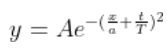(a) Write the dimensions of A, a and T. (b) Find the wave speed. (c) In which direction is the wave travelling? (d) Where is the maximum of the pulse located at t = T? At t = 2 T?

Solution:

(a) dimensions of A, a and T

[A] = [L] [a] = [L] and

[T] = [T]

(b) Find the wave speed:

Wave speed = v = λ/T = a/T

(c)

The whole structure depends upon the exponent.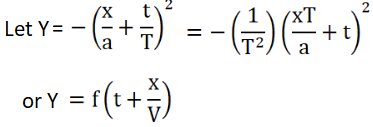Now,

Case 1: If y = f(t-x/v), then wave is travelling in positive direction.

Case 2: If y = f(t + x/v), x/v), then wave is travelling in negative direction.

(d) wave speed = v = a/T

The maximum pulse at t = T is (a/T) x T = a -> negative x-axis

and maximum pulse at t = 2T is (a/T) x 2T = 2a -> along negative x-axis

So, the wave travels in negative x-axis direction.

Question 3: Figure (below) shows a wave pulse at t = 0. The pulse moves to the right with a speed of 10 cm s−1. Sketch the shape of the string at t = 1 s, 2 s and 3 s.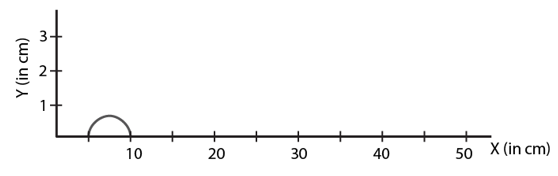Solution:

Using relation: x = vt

At t = 1 s the pulse will be at 10 cm.

At t = 2 s the pulse will be, x = 2(10) cm = 20 cm

At t = 3 s the pulse will be, x = 3(10)cm = 30 cm

Question 4: A pulse travelling on a string is represented by the function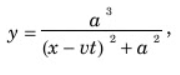where a = 5 mm and υ = 20 cm s−1. Sketch the shape of the string at t = 0, 1 s and 2 s. Take x = 0 in the middle of the string.

Solution:

y = a3/(x2+a2)

For maximum, dy/dx = 0

=> x = vt

again dx/dt = v

At t = 0 s, x = 0 cm

At t = 1 s, x = 20 cm

At t = 2 s, x = 40 cm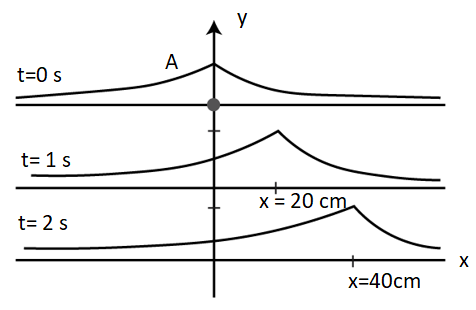Question 5: The displacement of the particle at x = 0 of a stretched string carrying a wave in the positive x-direction is given by f(t) = A sin(t/T). The wave speed is υ. Write the wave equation.

Solution: AT x = 0, f(t) = a sin(t/T)

=> wavelength = λ = vT

So, general equation of wave,

Y = A sin((t/T) – (x/vT))

Question 6: A wave pulse is travelling on a string with a speed υ toward the position X-axis. The shape of the string at t = 0 is given by g(x) = A sin (x/a), where A and a are constants.

(a) What are the dimensions of A and a?

(b) Write the equation of the wave for a general time t, if the wave speed is υ.

Solution:

(a) dimensions of A and a

[A]=[L] and [a]=[L]

(b) wave velocity is v (given). So, the time period will be,

T = λ/v

Here λ = a

=> T = a/v

Therefore, Y = sin(x/λ – t/T)

= A sin (x/a – vt/a)

= A sin [(x-vt)/a]

Question 7: A wave propagates on a string in the positive x-direction at a velocity υ. The shape of the string at t = t0 is given by g(x, t0) = A sin(x/a). Write the wave equation for a general time t.

Solution:

The general equation:

Y = A sin(x/λ – t/T)

Here λ = a also T = a/v

Y = A sin(x/a – vt/a)

=> A sin(x/a) = A sin(x/a + vt_0/a)

To sustain equality, the equation must be,

Y = A sin(x/a – vt/a + vt_0/a)

Question 8: The equation of a wave travelling on a string is

y = (0.10 mm) sin[(31.4 m−1) x+ (314 s−1) t].

(a) In which direction does the wave travel?

(b) Find the wave speed, the wavelength and the frequency of the wave.

(c) What is the maximum displacement and the maximum speed of a portion of the string?

Solution:

The equation of a wave travelling on a string:

y = (0.10 mm) sin[(31.4 m−1) x+ (314 s−1) t].

We know, The structure of the equation is y = A sin(kx + ωt)

(a) Negative x -direction.

(b) k = 31.4 m−1

=> 2π/λ = 3.14

or λ = 20 cm

Again, ω = 314 s−1

=> 2πf = 314

=> f = 50 sec−1

Therefore, wave speed = v = λf = 20 x 50 = 1000 cm/s

(c) Max displacement = 0.10 mm

Max. velocity = aω = (0.1) x 10−1 x 314 = 3.14 cm/sec

Question 9: A wave travels along the position x-direction with a speed of 20 m s−1. The amplitude of the wave is 0.20 cm and the wavelength 2.0 cm. (a) Write a suitable wave equation which describes this wave. (b) What is the displacement and velocity of the particle at x = 2.0 cm at time t = 0 according to the wave equation written? Can you get different values of this quantity if the wave equation is written in a different fashion?

Solution:

Here, λ = 2 cm, V = 2.0 m/s and A = 0.20 cm

(a) Equation of wave along the x-axis

y = A sin(kx – wt)

k = 2π/λ = π cm−1

and T = λ/v = 2/2000 = 10-3 sec

This implies, ω = 2π/T = 2π 103 sec

So, the wave equation is,

y = (0.2) sinπ x – (2π 103 )t

(b) At x = 2 cm and t = 0

y = (0.2)sin(π/2) = 0

Therefore, particle velocity, v = rω cos(πx) = 0.2 x 2000π x cos 2π = 400π

= 400 x 3.14

= 4π m/s

Question 10: A wave is described by the equation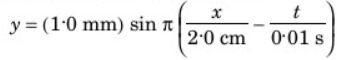(a) Find the time period and the wavelength?

(b) Write the equation for the velocity of the particles. Find the speed of the particle at x = 1.0 cm at time t = 0.01 s.

(c) What are the speeds of the particles at x = 3.0 cm, 5.0 cm and 7.0 cm at t = 0.01 s?

(d) What are the speeds of the particles at x = 1.0 cm at t = 0.011, 0.012 and 0.013 s?

Solution:

(a) T = 2×0.01 sec = 20 min

λ = 2×2 = 4 cm

(b) v = dy/dt = d/dt [sin2π(x/4 – t/0.02)]

= – cos 2π (x/4 – t/0.02) x 1/0.02

=> v = -50 cos 2π (x/4 – t/0.02)

At x = 1 and t = 0.01 sec, v = -50 cos 2(1/4-1/2) = 0

(c)

At x = 3 cm, t = 0.01 sec

v = -50 cos 2π(3/4 – 1/2) = 0

At x = 5 cm, t = 0.01 sec, v = 0

At x = 7 cm and t = 0.011 sec, v =0

At x = 1 cm, and t = 0.011 sec

v = -50 cos 2π[1/4 – (0.011/0.02)]

= -9.7 cm/sec

Question 11: A particles on a stretched string supporting a travelling wave takes 50 ms to move from its mean position to the extreme position. The distance between two consecutive particles, which are at their mean positions, is 20 cm. Find the frequency, the wavelength and the wave speed.

Solution:

frequency of vibration = f = 1/T = 50 Hz

Any two neighbouring mean positions always remain at half of the wave length, λ = 4 cm

Now, wave speed = v = λf = 2 m/s

Question 12: Figure (below) shows a plot of the transverse displacements of the particles of a string at t = 0 through which a travelling wave is passing in the positive x-direction. The wave speed is 20 cm s−1. Find (a) the amplitude, (b) the wavelength, (c) the wave number and (d) the frequency of the wave.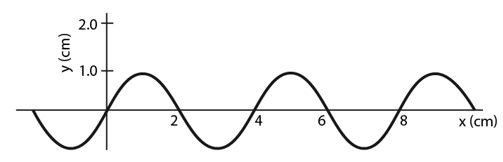Solution:

V = 200 m/s (given)

(a) amplitude = A = 1 mm

(b) the wavelength = λ= 4 cm

(c) the wave number = n = 2π/λ = 1.57 cm−1

(d) the frequency of the wave = f = 1/T = (26/λ)/20 = 5 Hz

Where T = λ/v

Question 13: A wave travelling on a string at a speed of 10 m s−1 causes each particle of the string to oscillate with a time period of 20 ms.

(a) What is the wavelength of the wave?

(b) If the displacement of a particle is 15 mm at a certain instant, what will be the displacement of a particle 10 cm away from it at the same instant?

Solution:

(a) v = λ/T

or λ = vt = 20 cm

(b) Phase shift difference = (2π/λ)x = 2π/20 x 10 = π rad

y_1 = a sin(ωt – kx)

=> 1.5 = a sin(ωt – kx)

the displacement of a particle at x = 10 cm

y_2 = a sin(ωt – kx + π)

=> -a sin(ωt – kx) = -1.5 mm

Therefore, displacement of a particle is -1.5 mm

Question 14: A steel wire of length 64 cm weights 5 g. If it is stretched by a force of 8 N, what would be the speed of a transverse wave passing on it?

Solution:

Mass = 5g, Length = 64cm and Force = 8 N (given)

So, density= ρ = (5/64) g/cm

Now, F = ρv2

or v2 = 8 x (64 cm/5g)

or v = 32 m/s

Question 15: A string of length 20 cm and linear mass density 0.40 g cm−1 is fixed at both ends and is kept under a tension of 16 N. A wave pulse is produced at t = 0 near an end as shown in figure (below), which travels towards the other end.

(a) When will the string have the shape shown in the figure again? (b) Sketch the shape of the string at a time half of that found in part (a).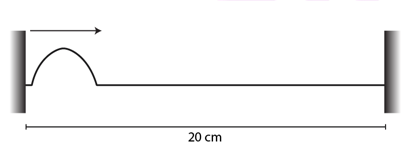Solution:

(a) velocity of wave = v2 = T/m = (16×105)/0.4

or v = 2000 cm/s

Time taken to reach other end = 20/2000 = 0.01 sec

Time taken to see the pulse again in the original position = 0.01 x 2 = 0.02 s

(b) At t = 0.01 s, there will be a though at the right end as it is reflected.

Question 16: A string of linear mass density 0.5 g cm−1 and a total length 30 cm is tied to a fixed wall at one end and to a frictionless ring at the other end (figure below). The ring can move on a vertical rod. A wave pulse is produced on the string which moves towards the ring at a speed of 20 cm s−1. The pulse is symmetric about its maximum which is located at a distance of 20 cm from the end joined to the ring.

(a) Assuming that the wave is reflected from the ends without loss of energy, find the time taken by the string to regain its shape. (b) The shape of the string changes periodically with time. Find this time period.

(c) What is the tension in the string?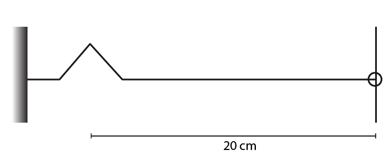Solution:

(a) The distance travelled by the wave = 20+20 = 40 cm

time = t = x/v = 40/20 = 2 sec

(b) The string regains its original shape after completing a periodic distance i.e. (30+30) cm = 60cm.

time period = 60/20 = 3 sec

(c) frequency = n = (1/3) sec−1

n = (1/2I)√(T/m)

m = mass per unit length = 0.5 g/cm

=> (1/3) = 1/(2×30) x √(T/0.5)

=> T = 2 x 10-3 N

Question 17: Two wires of different densities but same area of cross section is soldered together at one end and are stretched to a tension T. The velocity of a transverse wave in the first wire is double of that in the second wire. Find the ratio of the density of the first wire to that of the second wire.

Solution: Let v1 and v2 are the velocities of wires.

ρ1 and ρ2 are the respective densities.

Therefore, T1 = T2

=> ρ1 v12 = ρ2 v22

or ρ12 = v22/v12

Given that, V1 = 2V2

ρ12 = v22/4v22 = 1/4

or ρ12 = 0.25

Question 18: A transverse wave described by

y = (0.02 m) sin(1.0 m−1) x + (30 s−1)t]

propagates on a stretched string having a linear mass density of 1.2 × 10−4 kg m-1. Find the tension in the string.

Solution: A transverse wave described by

y = (0.02 m) sin(1.0 m−1) x + (30 s−1)t]

Speed = v = ω/k

here, ω = 30 sec−1 and k = 1 m−1

=> v = 30 m/s

But T = ρv2

= 1.2 x 90 x 10-4 N

or T = 0.108 N

Question 19: A travelling wave is produced on a long horizontal string by vibrating and end up and down sinusoidally. The amplitude of vibration is 1.0 cm and the displacement becomes zero 200 times per second. The linear mass density of the string is 0.10 kg m−1 and it is kept under a tension of 90 N.

(a) Find the speed and the wavelength of the wave.

(b) Assume that the wave moves in the position x-direction and at t = 0, the end x = 0 is at its positive extreme position. Write the wave equation.

(c) Find the velocity and acceleration of the particle at x = 50 cm at time t = 10 ms.

Solution:

Amplitude = A = 1 cm, tension = T = 90N, frequency = f = 200/2 = 100 Hz and mass=m = 0.1 kg/m

(a)

v = √(T/ρ)

= √(90/0.1) = 30 m/s

Again, v = λf = 30 cm

(b) y = 10 cos 2π[x/30 – t/0.01]

At t = 0 and x = 0, it has maxima, consists a phase of π/2

=> y = (1) sin[2πx/30 – 2πt/0.01 + π/2]

the required equation is, y = (1) cos[2πx/30 – 2πt/0.01]

(c) The velocity of the particle,

y’ = -(1) (2π/0.01) sin[2πx/30 – 2πt/0.01]

At x = 50 cm and t = 10 s

y’ = -5.4 m/s

Now the acceleration is :

y” = -(1) (2π/0.01)^2 cos[2πx/30 – 2πt/0.01]

At x = 50 cm and t = 10 s

y” = 2 km/s2

Question 20: A string of length 40 cm and weighing 10 g is attached to a spring at one end and to a fixed wall at the other end. The spring has a spring constant of 160 N m−1 and is stretched by 1.0 cm. If a wave pulse is produced on the string near the wall, how much time will it take to reach the spring?

Solution:

Here l = 40 cm , spring constant = k = 160 N/m and mass = 10 g

Mass per unit length = m = 10/40 = (1/4) g/cm

Now, deflection = x = 1 cm = 0.01 m

=> T = kx = 1.6 N = 16 x 104 dyne

Also, v = √(T/m) = 8 x 102 cm/s = 800 cm/s

Therefore, time taken by the pulse to reach the spring:

t = 40/800 = 0.05 sec

Question 21: Two blocks each having a mass of 3.2 kg are connected by a wire CD and the system is suspended from the ceiling by another wire AB (figure below). The linear mass density of the wire AB is 10 g m−1 and that of CD is 8 g m−1. Find the speed of a transverse wave pulse produced in AB and in CD.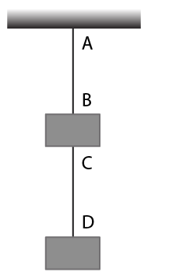Solution:

Force due to gravity on AB:

TAB = (3.2 + 3.2)9.8 N = 62.72 N

Force due to gravity on CD:

TAB = 3.2 x 9.8 = 31.36 N

The velocities are:

vAB = √(TABAB) = 79 m/s

and

vCD = √(TCDCD) = 63 m/s

Question 22: In the arrangement shown in figure (below), the string has a mass of 4.5 g. How much time will it take for a transverse disturbance produced at the floor to reach the pulley? Take g = 10 m s−2.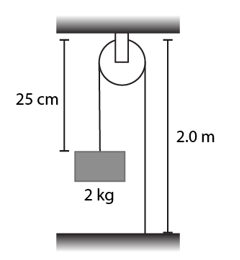Solution:

Mass density = ρ = (0.0045/2.25) kg/m

force on the string = T = 20 N

Now, speed of the wave = v = √(T/ρ) = 100 m/s

Therefore, time taken = t = 2.25/100 = 0.02 sec

Question 23: A 4.0 kg block is suspended from the ceiling of an elevator through a string having a linear mass density of 19.2 × 10−3 kg m−1. Find the speed (with respect to the string) with which a wave pulse can proceed on the string if the elevator accelerates up at the rate of 2.0 m s−2. Take g = 10 m s−2.

Solution:

T = ma + mg = (4×2 + 4×10) = 48 N

And, speed = v = √(T/ρ) = 50 m/s

Question 24: A heavy ball is suspended from the ceiling of a motor car through a light string. A transverse pulse travels at a speed of 60 cm s−1 on the string when the car is at rest and 62 cm s−1 when the car accelerates on a horizontal road. Find the acceleration of the car. Take g = 10 m s−2.

Solution:

Tension = T = mg

Speed = v1 = √(mg/ρ)

At motion:

Tension = T = m√(a2+g2) and

Speed = v2 = √[(m√(a2 + g2))/ρ]

Now,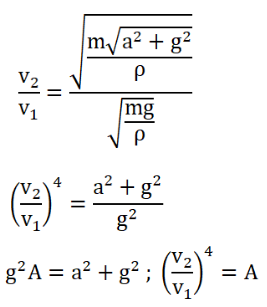or (A – 1)g2 = a2

a2 = 0.140 x 100

or a = 3.74 m/s2

Question 25: A circular loop of string rotates about its axis on a frictionless horizontal plane at a uniform rate so that the tangential speed of any particle of the string is υ. If a small transverse disturbance is produced at a point of the loop, with what speed (relative to the string) will this disturbance travel on the string?

Solution:

R = Radius of the loop, m = mass per unit length of the string

ω = angular velocity, V = linear velocity of the string

The force for a small potion in the ring:

dF = (mRdθ)ω2 R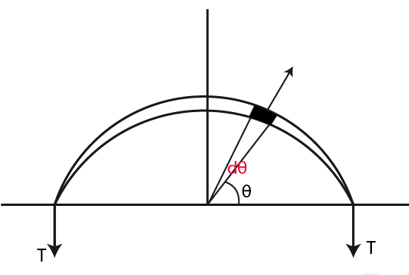dF = 2 (mRdθ)ω2 R sin θ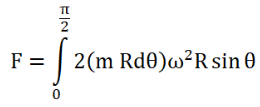or F = 2mR2 ω2

But whole of this process was for half of the ring:

2T = 2mR2 ω2

Or T = mR2 ω2

Now, velocity, v = √(T/m) = Rω

Which is the speed of the disturbance.

Question 26: A heavy but uniform rope of length L is suspended from a ceiling. (a) Write the velocity of a transverse wave travelling on the string as a function of the distance from the lower end. (b) If the rope is given a sudden sideways jerk at the bottom, how long will it take for the pulse to reach the ceiling? (c) A particle is dropped from the ceiling at the instant the bottom end is given the jerk. Where will the particle meet the pulse?

Solution:

(a) Downward weight for the element = (mx)g = Tension in the string of upper part

velocity of transverse vibration = v = √(T/m) = √(mglm) = √gx

(b) For small displacement dx, dt = dx/√gx

Total time: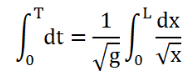= √(4L/g)

(c) Suppose, it will meet the pulse after y distance.

To get the in between time, we integrate,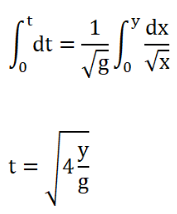Therefore, distance travelled by the particle in this time is (L-y)

We know the relation, S = ut + (1/2)gt2

=> L – y(1/2)g x √(4y/g) 2 at u = 0

=> L – y = 2y

=> y = L/3, which shows that, the particle meet at distance L/3 from the lower end.

Question 27: Two long strings A and B, each having linear mass density 12 × 10−2 kg m−1, are stretched by different tensions 48 N and 75 N respectively and are kept parallel to each other with their left ends at x = 0. Wave pulses are produced on the strings at the left ends at t = 0 on string A and at t = 20 ms on string B. When and where will the pulse on B overtake that on A?

Solution:

Suppose v and v’ are wave speeds in string A and B respectively.

T = 4.8 and m = 1.2 x 10-2 and T’ = 7.5

Now,

v = √(T/m) = 20 m/s and

v’ = √(T’/m) = 20 m/s = 25 m/s

t = 0 in string A,

t_1 = 0+20 ms = 20 x 10-3 = 0.02 sec

In 0.02 sec A has travelled 20 x 0.02 = 0.4 mt

relative speed between A and B = 25-20 = 5 m/s

Time taken for B for overtake A = s/v = 0.4/5 = 0.08 sec

Question 28: A transverse wave of amplitude 0.50 mm and frequency 100 Hz is produced on a wire stretched to a tension of 100 N. If the wave speed is 100 m s−1, what average power is the source transmitting to the wire?

Solution:

Average power of the source = P = 2π2mva2f2…(1)

v = √(T/m) = 100 m/s and m = 0.01 kg/m

r = 0.5 x 10-3 and f = 100

(Given)

On substituting the values, (1)=> P = 49 mW

Question 29: A 200 Hz wave with amplitude 1 mm travels on a long string of linear mass density 6 g m−1 kept under a tension of 60 N. (a) Find the average power transmitted across a given point on the string.

(b) Find the total energy associated with the wave in a 20 m long portion of the string.

Solution:

Here A = 1 mm = 10-3 mA, m = 6 g/m = 6 x 10-3 kg/m

F = 200 Hz and T = 60 N

(a) Average power of the source = P = 2π2mvA2f2 = 0.47 W

(b) Length of string = 2m

And t = 2/100 = 0.02 sec

So, Energy = 2π2mvtA2f2 = 9.46 mJ

Question 30: A tuning fork of frequency 440 Hz is attached to a long string of linear mass density 0.01 kg m−1 kept under a tension of 49 N. The fork produces transverse waves of amplitude 0.50 mm on the string.

(a) Find the wave speed and the wavelength of the waves.

(b) Find the maximum speed and acceleration of a particle of the string.

(c) At what average rate is the tuning fork transmitting energy to the string?

Solution: Given, m = 0.01 kg/m, T = 49N, r = 0.5 x 10-3 m and f = 440 Hz

(a) Let the wavelength of the wave be λ.

Speed of the transverse wave = v = √(T/m) = √(49/0.01) = 70 m/s

Also, v = f/λ

or λ = f/v = 70/440 = 16 cm

(b) y = A sin(ωt – kx)

Therefore, v = dy/dt = Aω cos(ωt – kx)

Now,

vmax = (dy/dt) = Aw

= (0.50) x 10-3 x 2π x 440

= 1.381 m/s

and

a = d2y/dt2

=> a = -Aω2 sin(ωt – kx)

amax = -Aω2

= 0.50 x 10-3 x 4π2 x 4402

= 3.8 km/s2

(c) Average rate = p = 2π2vA2f2

= 2 x 10 x 0.01 x 70 x (0.50×10-3) 2 x 4402

= 0.67 W

Question 31: Two waves, travelling in the same direction through the same region, have equal frequencies, wavelengths and amplitudes. If the amplitudes of each wave is 4 mm and the phase difference between the waves is 90°, what is the resultant amplitude?

Solution: Consider equation of waves:

y’ = r sin ωt and y” = r sin (ωt + π/2)

Now, y = y’ + y”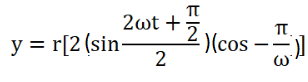Or y = √2 r sin(ωt + π/4)

The amplitude is 4√2 mm

Question 32: Figure (below) shows two wave pulses at t = 0 travelling on a string in opposite directions with the same wave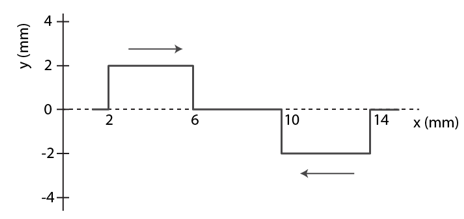Solution:

Distance travelled by any classical wave = s = vt

At t = 4 => s = 4 x 10-3 x 50 x 10 = 2 mm

At t = 6 => s = 6 x 10-3 x 50 x 10 = 3 mm

At t = 8 => s = 8 x 10-3 x 50 x 10 = 4 mm

At t = 12 => s = 12 x 10-3 x 50 x 10 = 6 mm

Question 33: Two waves, each having a frequency of 100 Hz and a wavelength of 2.0 cm, are travelling in the same direction on a string. What is the phase difference between the waves

(a) if the second wave was produced 0.015 s later than the first one at the same place,

(b) if the two waves were produced at the same instant but the first one was produced a distance 4.0 cm behind the second one?

(c) If each of the waves has an amplitude of 2.0 mm, what would be the amplitudes of the resultant waves in part (a) and (b)?

Solution:

(a) Wave speed = wave length × wave frequency

v = 100 x 0.02 = 2 m/s

In 0.015 sec, the path travelled by wave,

x = 0.015 x 2 = 0.03 m

The phase difference:

φ = (2πx)/λ = (2π)/0.02 x 0.03 = 3π

(b) Again, for path difference, x = 0.04 m

φ = 4π

(c) Two waves have same amplitude if their frequency and wavelength are same.

Now, consider two wave equations, y’ = r sin ωt and y” = r sin (ωt + φ)

=> y = y’ + y”

= 2r sin (ωt + φ/2)cos (φ/2)

Therefore, resultant amplitude, A = 2r cos(φ/2)

For A = 0, φ = 3π

For A = 4, φ = 4π

Question 34: If the speed of a transverse wave on a stretched string of length 1 m is 60 m s−1, what is the fundamental frequency of vibration?

Solution:

Fundamental frequency = f = v/2L = 30 Hz

Question 35: A wire of length 200 m is stretched to a tension of 160 N. If the fundamental frequency of vibration is 100 Hz, find its linear mass density.

Solution:

Fundamental frequency = f = v/2L = 1/2L x √(T/m) = 1 g/m

Question 36: A steel wire of mass 40 g and length 80 cm is fixed at the two ends. The tension in the wire is 50 N. Find the frequency and wavelength of the fourth harmonic of the fundamental.

Solution:

Fundamental frequency = f = v/2L = 1/2L x √(T/m) = 62.5 Hz

frequency of 4th harmonic = F4 = 4 x 62.5 = 250 Hz

Now, v = F4 λ4

or λ4 = 250/v = 40 cm

Question 37: A piano wire weighing 6.0 g and having a length of 90.0 cm emits a fundamental frequency corresponding to the “Middle C” (ν = 261.63 Hz). Find the tension in the wire.

Solution:

Fundamental frequency = f = (1/2L)√(T/m)

or f = √150 T

Also, f = 261.63 Hz (given)

=> 261.63 = √150 T

=> T = 1480 N (approx.)

Question 38: A sonometer wire having a length of 1.50 m between the bridges vibrates in its second harmonic in resonance with a tuning fork of frequency 256 Hz. What is the speed of the transverse wave on the wire?

Solution:

Fundamental frequency of First Harmonic = 256/2 = 128 Hz

Here, Second harmonic= 2 × First Harmonic

When the fundamental wave is produced, λ/2 = 1.5

=> λ = 3 m

speed of the wave = f λ = 128 x 3 = 384 m/s

Question 39: The length of the wire shown in figure (below) between the pulley is 1.5 m and its mass is 12.0 g. Find the frequency of vibration with which the wire vibrates in two loops leaving the middle point of the wire between the pulleys at rest.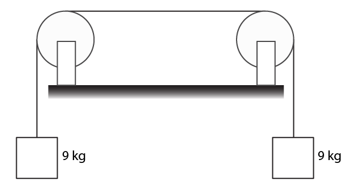Solution:

Mass of the wire = 12 gm

Length of the wire between two pulleys (L) = 1.5 m

so, Mass per unit length = m = (12/1.5) g/m = 8 x 10-3 kg/m

Tension in the wire = T = 9g = 90 N

Now, Fundamental frequency = f’ = (1/2L) √(T/m)

Second harmonic = 2(First Harmonic )

=> f = 2 f’ = (1/1.5) x √(90/8 x 10^-3)

= 70.7 Hz

Question 40: A one-metre long stretched string having a mass of 40 g is attached to a tuning fork. The fork vibrates at 128 Hz in a direction perpendicular to the string. What should be the tension in the string if it is to vibrate in four loops?

Solution:

Using relation, L = nλ/2

Here n = 4 and L = 1 m

=> λ = 0.5

Also, v = fλ = √(T/m)

=> T = f2λ2 m = 1282 x 0.52 x 40 x 10-3

= 164 N

Question 41: A wire, fixed at both ends is seen to vibrate at a resonant frequency of 240 Hz and also at 320 Hz.

(a) What could be the maximum value of the fundamental frequency?

(b) If transverse waves can travel on this string at a speed of 40 m s−1, what is its length?

Solution:

(a) Two frequencies are v1 = 240 Hz and ν2 =320 Hz

So, Maximum fundamental frequency = ν2 – ν1

​= 320 − 240 = 80 Hz

(b) Given v = 40 m/s

=> L x 80 = 0.5 x 40

=> L = 0.25 m

Question 42: A string, fixed at both ends, vibrates in a resonant mode with a separation of 2.0 cm between the consecutive nodes. For the next higher resonant frequency, this separation is reduced to 1.6 cm. Find the length of the string.

Solution:

Let n be the frequency, L is length of the string and λ be the distance between two consecutive nodes.

Therefore, L = nλ

for next higher frequency, say (n+1) the distance between two consecutive nodes is λ′, then

L = (n+1)λ’

Equating Equations, we get

nλ = (n+1)λ’

or λ’ = n(λ – λ’)

Here λ = 2 cm and λ’ = 1.6 cm

On putting values,

n = 4

=>L = 4×2 = 8 cm

Question 43: A 660 Hz tuning fork sets up vibration in a string clamped at both ends. The wave speed for a transverse wave on this string is 220 m s−1 and the string vibrates in three loops.

(a) Find the length of the string.

(b) If the maximum amplitude of a particle is 0.5 cm, write a suitable equation describing the motion.

Solution :

f = 660 Hz and v = 220 m/s

Wave length = λ = v/f = 1/3 m

(a) Number of loops = n =3

Therefore, L = (nλ)/2 = (3/2) x (1/3) = 1/2 m = 50 cm

(b) resultant wave equation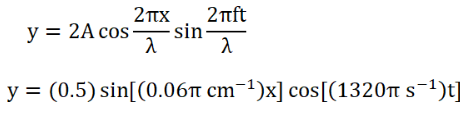[On Substituting the values of λ,f and A]

Question 44: A particular guitar wire is 30.0 cm long and vibrates at a frequency of 196 Hz when no finger is placed on it. The next higher notes on the scale are 220 Hz, 247 Hz, 262 Hz and 294 Hz. How far from the end of the string must the finger be placed to play these notes?

Solution:

We know that, f ∝ 1/l or f = v/l (where v = constant for a medium)

l1 = 30 cm or 0.3 m (given)

f1 = 196 Hz and f2 = 220 Hz (given)

Now, f1/f2 = l2/l1

=> l2 = 26.7 cm

Again, f3 = 247 Hz

so, f3/f1 = l1/l3 = 0.3/l3

=> l3 = 0.224 m = 22.4 cm

in same way, we have l4 = 20 cm

Question 45: A steel wire fixed at both ends has a fundamental frequency of 200 Hz. A person can hear sound of maximum frequency 14 kHz. What is the highest harmonic that can be played on this string which is audible to the person?

Solution:

Fundamental frequency = fo =200 Hz

nth harmonic = f’ = n × fundamental frequency

and frequency of the highest harmonic = 14 kHz = 14000 Hz

Now, f’/fo = 14000/200

nfo/fo = 70

=> n = 70

The highest audible to man is 70th harmonic.

Question 46: Three resonant frequencies of the string are 90, 150 and 210 Hz. (a) Find the highest possible fundamental frequency of vibration of this string.

(b) Which harmonics of the fundamental are the given frequencies?

(c) Which overtones are these frequencies?

(d) If The length of the string is 80 cm, what would be the speed of a transverse wave on this string?

Solution:

(a) gcd of 90,150 and 210 is 30

So, f = 30 Hz

(b) Let f1, f2 and f3 are Three resonant frequencies of the string.

f1 = 3f, f2 = 5f and f3 = 7f

(c) nth overtone is (n+1)th frequency.

So, 3f is 2nd overtone and 3rd harmonic.

5f is 4th overtone and 6th harmonic.

and 7f is 6th overtone and 7th harmonic.

(d) We know, f1 = (3/2)v

so, 90 = (3/2×80) k

=> k = 48 m/s

Question 47: Two wires are kept right between the same pair of supports. The tensions in the wires are in the ratio 2:1, the radii are in the ratio 3:1 and the densities are in the ratio 1 : 2. Find the ratio of their fundamental frequencies.

Solution: The ratio of mass per unit length of the wires:

p12 x r12/r22 = (1/2) x (9/1)= 9/2

Fundamental frequency of wire = (1/2l) √(T/μ)

Thus, f1/f2 = 2:3

Question 48: A uniform horizontal rod of length 40 cm and mass 1.2 kg is supported by two identical wires as shown in figure (below). Where should a mass of 4.8 kg be placed on the rod so that the same tuning fork may excite the wire on left into its fundamental vibrations and that on right into its first overtone? Take g = 10 m s−2.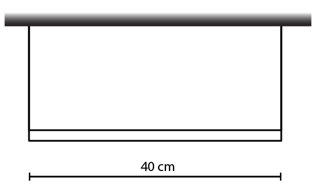Solution:

We know, f = (1/2L) √(T/m)

Given, fd = 2f2

SO, √(T1/T2) = 2

Now, T1 + T2 = 48 + 12 = 60 N

By replacing the relations, T1 = 48 N and T2 = 12 N

Taking moment about a point, T2 x 0.4 = 48x + 12(0.2)

on solving above equation, we have x = 5 cm

Therefore, mass should be placed at a distance 5cm from the left end.

Question 49: Figure (below) shows an aluminium wire of length 60 cm joined to the steel wire of length 80 cm and stretched between two fixed supports. The tension produced is 40 N. The cross-sectional area of the steel wire is 1.0 mm2 and that of the aluminium wire is 3.0 mm2. What could be the minimum frequency of a tuning fork which can produce standing waves in the system with joint as a node? The density of aluminium is 2.6 gcm-3 and that of steel is 7.8 g cm-3.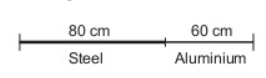Solution:

Calculate the mass per unit length of aluminium and steel wire using given values.

We know, ρ = M/V

M/l = ρA

Here, m = M/l

so, m = ρA

For aluminium:

Put the value into the formula

ma = 2.6 x 3 x 10-2 = 7.8 x 10-2 g/cm

For steel:

ms = 7.8 x 10-2 g/cm

Now, v = √(T/m)

Here, T = 40 N and m = 7.8 x 10-2 g/cm

=> v = 71.6 m/s

For minimum frequency, there would be maximum wavelength. And, for maximum wavelength, minimum number of loops are to be produced.

Wavelength = λ = 2d = 2 x 20 = 40 cm

The minimum frequency of a tuning fork :

f = v/λ

Given v = 71.6 m/s and λ = 0.4 m

=> f = 179 Hz

Question 50: A string of length L fixed at both ends vibrates in its fundamental mode at a frequency v and a maximum amplitude A.

(a) Find the wavelength and the wave number k.

(b) Take the origin at one end of the string and the X-axis along the string. Take the Y-axis along the direction of the displacement. Take t = 0 at the instant when the middle point of the string passes through its mean position and is going towards the positive y-direction. Write the equation describing the standing wave.

Solution:

Let L be the length of string.

Velocity of wave = v = √(T/m)

(a) wavelength = λ = velocity/frequency

=> λ = √(T/m) x 1/[(1/2L)√(T/m)] = 2L

Now, wave number = k = 2π/λ

= 2π/2L = π/L

(b) Equation of the stationary wave:

y = A cos (2πx/λ) sin (2πVt/λ)

As, v = V/2L

=> y = A cos (πx/L) sin (2π/vt)

Question 51: A 2 m-long string fixed at both ends is set into vibrations in its first overtone. The wave speed on the string is 200 m s−1 and amplitude is 0.5 cm. (a) Find the wavelength and the frequency. (b) Write the equation giving the displacement of different points as a function of time. Choose the x-axis along the string with the origin at one end and t = 0 at the instant when the point x = 50 cm has reached its maximum displacement.

Solution:

(a) Vibrating in first overtone means, n=2

We know, L = nλ/2

here, λ = L = 2 m

Again, f = v/λ = 100 Hz

(b) Suppose, the stationary wave equation:

y = 2A cos (2πx/λ) sin(2πvt/λ)

= 0.5 cos(2πx/2) sin(2π(200)t/λ)

= 0.5 cos[(πm-1)x] sin[(200)πs-1t]

Question 52: The equation for the vibration of a string, fixed at both ends vibrating in its third harmonic, is given by

y = (0.4 cm) sin[(0.314 cm−1)x] cos[(600π s−1)t]

(a) What is the frequency of vibration?

(b) What are the positions of the nodes?

(c) What is the length of the string?

(d) What is the wavelength and the speed of two travelling waves that can interface to give this vibration?

Solution:

The stationary wave equation

y = (0.4 cm) sin[(0.314 cm−1)x] cos[(600π s−1)t]

(a)frequency of vibration:

ω = 600π

So, 2πf = 600π

=> f = 300 Hz

(b) positions of the nodes:

sin(0.314x) = 0

=> 0.314x = kπ = k x 3.14

=> x = 10 K

Nodes are at 0, 10 cm, 20 cm and 30 cm.

(c) length of the string:

Length = 3λ/2 = 3 x 10 = 30 cm

(d) the wavelength and the speed of two travelling waves that can interface to give this vibration

wave equation = y = (0.4 cm) sin[0.314 x] cos(600πt]

=> λ = 20 cm

So, v = ω/k =6000 m/s = 60 m/s

Question 53: The equation of a standing wave, produced on a string fixed at both ends, is

y = (0.4 cm) sin(0.314 cm−1)x] cos[(600π s−1)t].

What could be the smallest length of the string?

Solution: Equation of standing wave

y = (0.4 cm) sin(0.314 cm−1)x] cos[(600π s−1)t].

Here, K = 0.314 = π/10

We know, λ = 2π/K = 20 cm

Now, L = (nλ/2)

For smallest length, n = 1

=> L = 20/2 = 10 cm

Question 54: A 40 cm wire having a mass of 3.2 g is stretched between two fixed supports 40.05 cm apart. In its fundamental mode, the wire vibrates at 220 Hz. If the area of cross section of the wire is 1.0 mm2, find its Young modulus.

Solution:

Strain = ΔI/I = 0.125 x 10-2 and f = 1/2L √(T/m)

=> T = 248.19 N

Now, stress = Tension/Area = 248.19 x 106

Therefore, Young modulus = stress/strain = 19852 x 108 N/m2